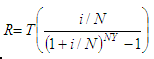# Term Deposit Interest Calculator

The calculator below helps you find out the amount required as a regular deposit to provide a stated future value in a specified time period. You have to enter the desired future value, the nominal interest rate, the number of deposits per year and the number of years. The calculator will return the needed amount for those regular deposits.

The calculation for regular deposits is based on the following formula:where:

R = amount of regular deposit
T = future value
N = number of deposits per year
Y = number of years
i = nominal interest rate

### Example to Calculate the deposit to achieve a Future Value

A good and nice person named Lois L. would like \$100,000 at the end of two years in a savings account. How much should Miss L. deposit monthly at 9% interest to achieve that goal?

Calculate Regular Deposits

Future Value = 100,000.00
Nominal Interest rate = 9.00
Nr. of deposits / yr = 12
Number of years = 2

Use the Deposit Calculator and you get...

Amount of regular deposit = 3,818.47

 Please, enable JavaScript codes on this page, otherwise the calculator won't work.Enter the needed values... (do not use commas) Future Value: Nominal Interest Rate: % Nr. of deposits / yr: Nr. of years: Amount of regular deposit:

From 'Term Deposit Interest Calculator' to home

From 'Term Deposit Interest Calculator' to Free Online Calculators

 Top Regular Deposits code in Matlab